# W is a rele that A linear transformation T from a vector space V into a...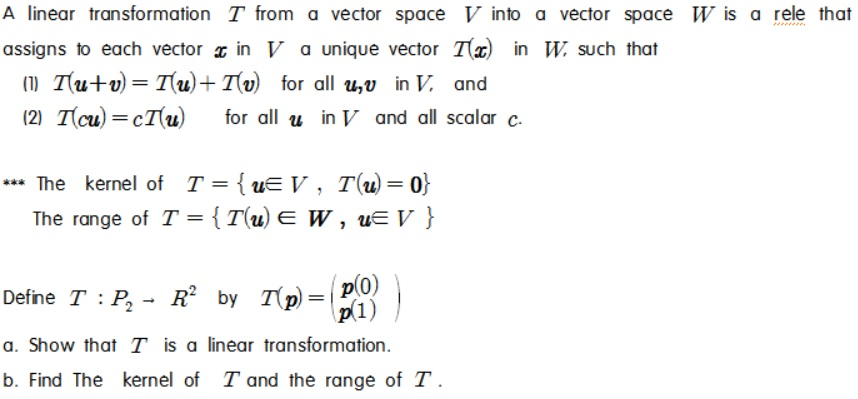W is a rele that A linear transformation T from a vector space V into a vector space assigns to each vector 2 in V a unique vector T() in W. such that (1) Tutu = Tu+Tv for all uv in V, and (2) Tſcu)=cT(u) for all u in V and all scalar c. *** The kernel of T = {UE V , T(U)=0} The range of T = {T(U) EW , ue V } Define T :P, - R K by T(m) = PO by 1 p = 0(1) a. Show that I is a linear transformation. b. Find The kernel of T and the range of T.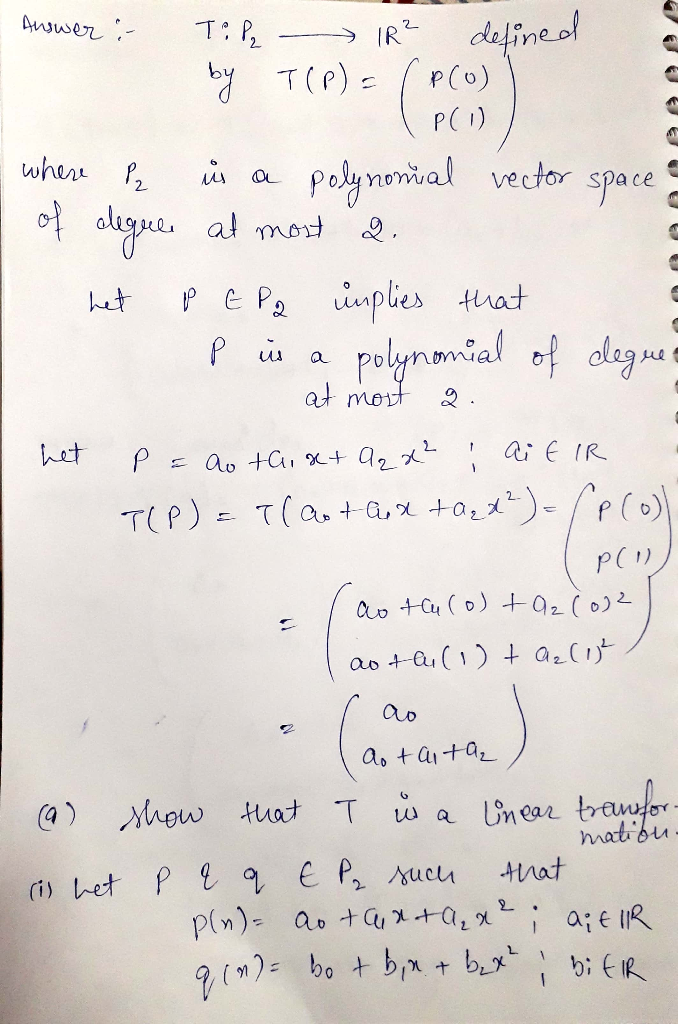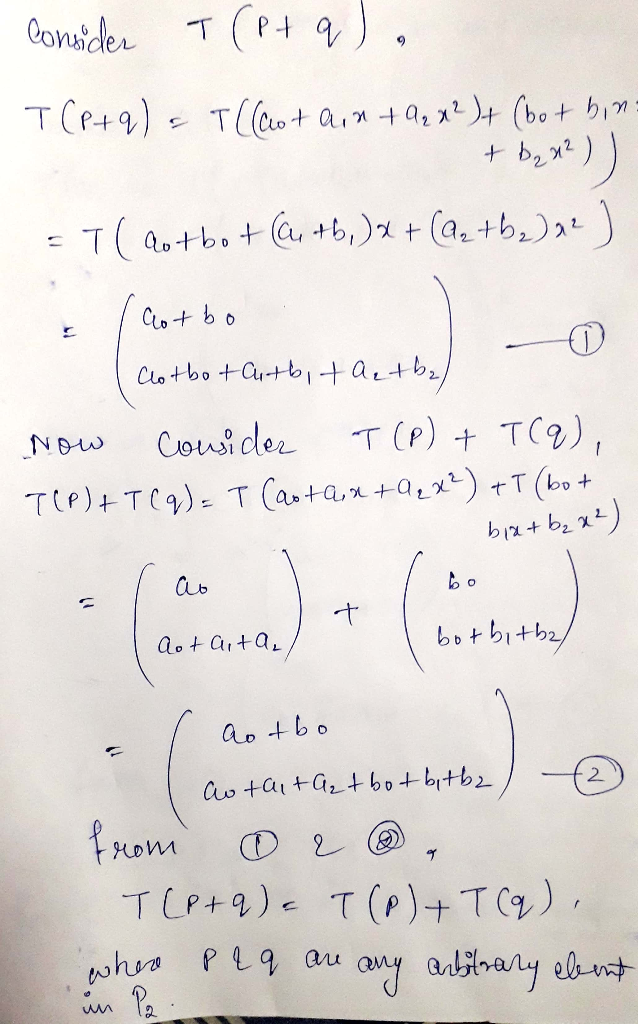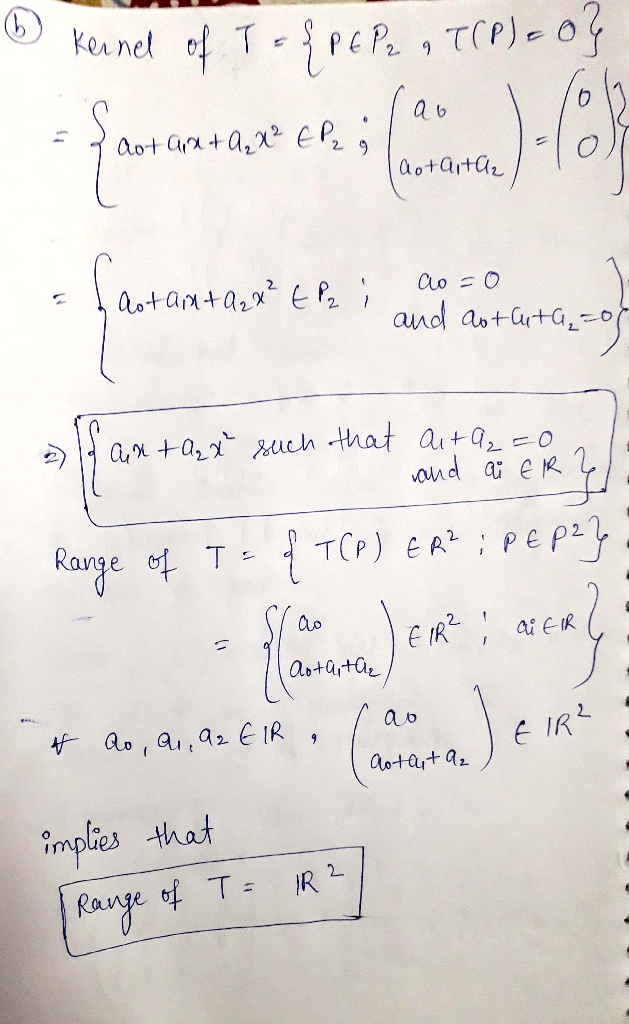##### Add Answer of: W is a rele that A linear transformation T from a vector space V into a...
Similar Homework Help Questions
• ### Problem 4. Let V be a vector space and let T : V → V and U : V → V be two linear transforinations...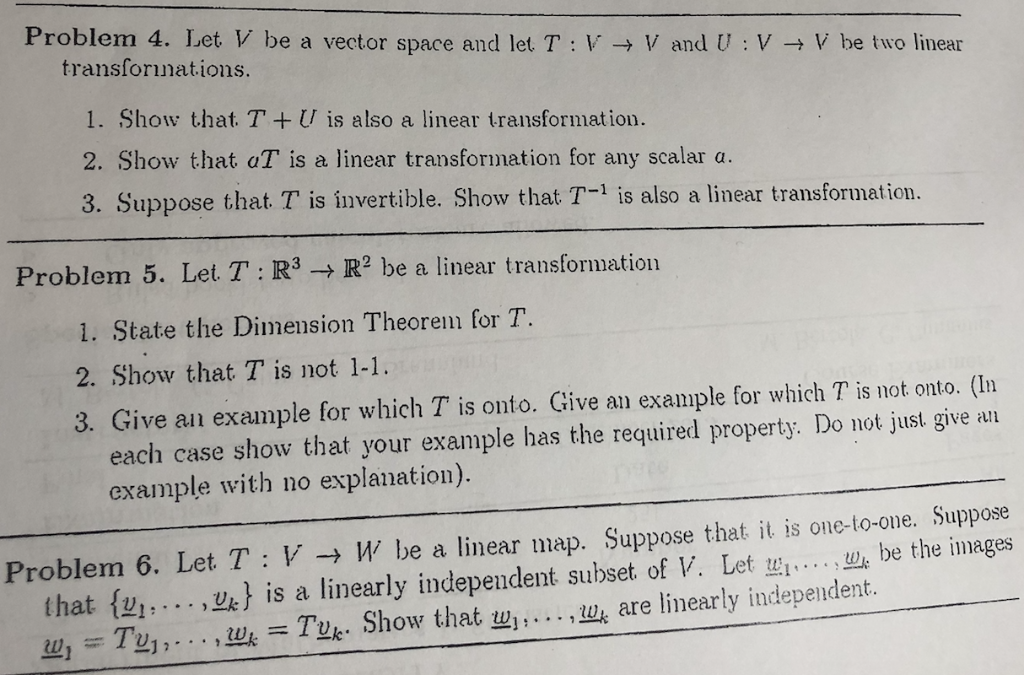I need the answer to problem 6 Clear and step by step please Problem 4. Let V be a vector space and let T : V → V and U : V → V be two linear transforinations 1. Show that. TU is also a linear transformation. 2. Show that aT is a linear transformation for any scalar a. 3. Suppose that T is invertible. Show that T-1 is also a linear transformation. Problem 5. Let T : R3 →...

• ### Let TRm → Rn be a linear transformation, and let p be a vector and S a set in R Show that the image of p + S under T i...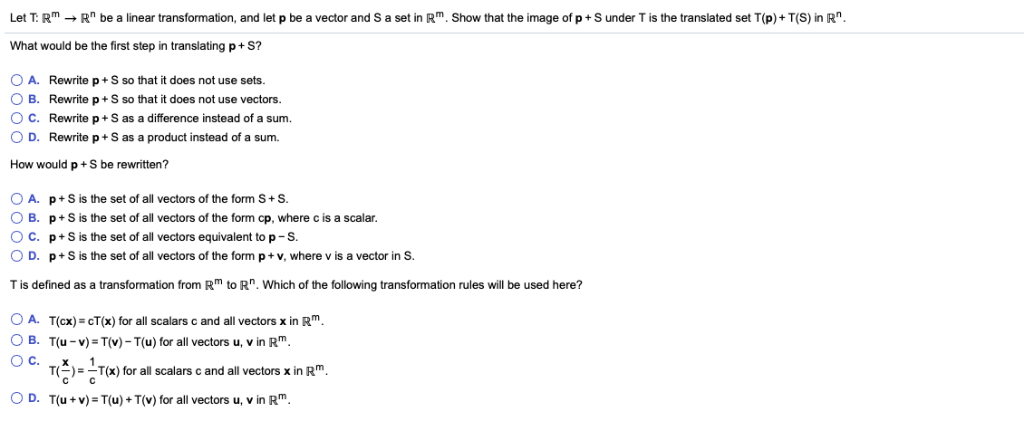Let TRm → Rn be a linear transformation, and let p be a vector and S a set in R Show that the image of p + S under T is the translated set T(p) + T(S) n R What would be the first step in translating p+ S? OA. Rewrite p+ S so that it does not use sets. O B. Rewrite p+S so that it does not use vectors O c. Rewrite p + S as a difference...

• ### Find an example of a vector space V, and a linear transformation T : V +...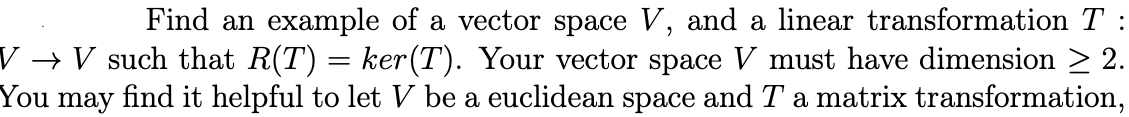Find an example of a vector space V, and a linear transformation T : V + V such that R(T) = ker(T). Your vector space V must have dimension > 2. You may find it helpful to let V be a euclidean space and T a matrix transformation,

• ### Let V be a finite-dimensional complex vector space and let T from V to V be...

Let V be a finite-dimensional complex vector space and let T from V to V be a linear transformation. Show that V is the direct sum of U and W where W and U are T-invariant subspaces and the restriction of T on U is nilpotent and the restriction of T on W is an isomorphism.

• ### Q4. Let T :V + W be a one-to-one linear transformation (i.e. an equation T(u) =...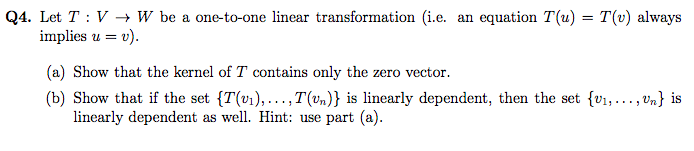Q4. Let T :V + W be a one-to-one linear transformation (i.e. an equation T(u) = T(v) always implies u = v). (a) Show that the kernel of T contains only the zero vector. (b) Show that if the set {T(v1), ...,T(un)} is linearly dependent, then the set {V1, ..., Un} is linearly dependent as well. Hint: use part (a).

• ### Q4. Let T :V + W be a one-to-one linear transformation (i.e. an equation T(u) =...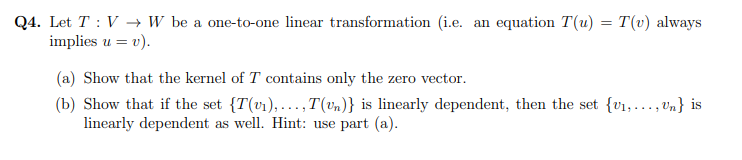Q4. Let T :V + W be a one-to-one linear transformation (i.e. an equation T(u) = T(v) always implies u = v). (a) Show that the kernel of T contains only the zero vector. (b) Show that if the set {T(v1),...,T(Un)} is linearly dependent, then the set {01, ..., Un} is linearly dependent as well. Hint: use part (a).

• ### Q4. Let T :V + W be a one-to-one linear transformation (i.e. an equation T(u) =...Q4. Let T :V + W be a one-to-one linear transformation (i.e. an equation T(u) = T(v) always implies u = v). (a) Show that the kernel of T contains only the zero vector. (b) Show that if the set {T(v1),...,T(Un)} is linearly dependent, then the set {01, ..., Un} is linearly dependent as well. Hint: use part (a).

• ### Q4. Let T :V + W be a one-to-one linear transformation (i.e. an equation T(u) =...Q4. Let T :V + W be a one-to-one linear transformation (i.e. an equation T(u) = T(v) always implies u = v). (a) Show that the kernel of T contains only the zero vector. (b) Show that if the set {T(v1),...,T(Un)} is linearly dependent, then the set {01, ..., Un} is linearly dependent as well. Hint: use part (a).

• ### Q4. Let T :V + W be a one-to-one linear transformation (i.e. an equation T(u) =...Q4. Let T :V + W be a one-to-one linear transformation (i.e. an equation T(u) = T(v) always implies u = v). (a) Show that the kernel of T contains only the zero vector. (b) Show that if the set {T(v1),...,T(Un)} is linearly dependent, then the set {01, ..., Un} is linearly dependent as well. Hint: use part (a).

• ### Q4. Let T :V + W be a one-to-one linear transformation (i.e. an equation T(u) =...Q4. Let T :V + W be a one-to-one linear transformation (i.e. an equation T(u) = T(v) always implies u = v). (a) Show that the kernel of T contains only the zero vector. (b) Show that if the set {T(v1),...,T(Un)} is linearly dependent, then the set {01, ..., Un} is linearly dependent as well. Hint: use part (a).

Free Homework App